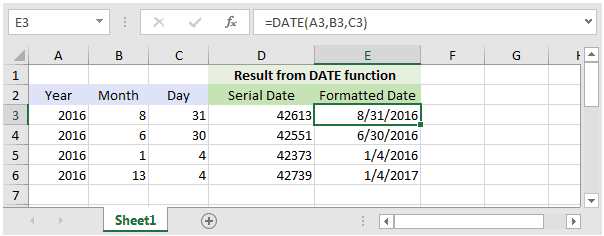## Excel Office

Excel How Tos, Tutorials, Tips & Tricks, Shortcuts

# DATE function: Description, Usage, Syntax, Examples and Explanation

## What is DATE function in Excel?

DATE function is one of Date and Time functions in Microsoft Office Excel that when you need to take three separate values and combine them to form a date.
Note: Microsoft Excel DATE function returns the serial date value for a date.

## Syntax of DATE function

The syntax for the DATE function in Microsoft Excel is:

`DATE( year, month, day )`

## DATE formula explanation

Year: A number that is between one and four digits that represents the year.
Month: A number representing the month value. If the month value is greater than 12, then every 12 months will add 1 year to the year value. This means that DATE(2016,13,4) is equal to DATE(2017,1,4) and DATE(2016,25,4) is equal to DATE(2018,1,4) … and so on.
Day: A number representing the day value. If the day value is greater than the number of days in the month specified, then the appropriate number of months will be added to the month value.
Also See:   How to generate series of dates increment by 1 year from a single start date in Excel

## Returns

The DATE function returns a serial date value. A serial date is how Excel stores dates internally and it represents the number of days since January 1, 1900.
If the year is greater than 9999, the DATE function will return the #NUM! error.

## Examples of DATE function

The example illustrated below shows DATE function result as an unformatted serial date in column D and column E show you the result as a formatted date in  `mm/d/yyyy`.Based on the Excel spreadsheet above, the following DATE examples would return:

```=DATE(A3,B3,C3)
Result: 42613  'Which can be formatted as "8/31/2016"

=DATE(A4,B4,C4)
Result: 42551  'Which can be formatted as "6/30/2016"

=DATE(A5,B5,C5)
Result: 42373  'Which can be formatted as "1/4/2016"

=DATE(A6,B6,C6)
Result: 42739  'Which can be formatted as "1/4/2017"```

Notice in the last example `=DATE(B6,B6,C6)` that the month value is 13 and larger than 12. In this example, 1 would be added to the year making it 2017. And the remainder of 1 would used as the month value.

Also See:   How to determine year is a leap year in Excel